Call us at (717) 735-9109, or email at support@rklesolutions.com

# Calling the Auto-Configurator as a Web Service in Sage ERP X3

When using the Configurator in Sage ERP X3, the Automate configurator function (FUNCFGAUT) can be used to automatically generate configuration. This function can be called from an X3 process, which can then be exposed as a Web Service. In the scenario below, the configurator is used to generate configurations from an outside web site, create the Item, Bill of Material and Routing in Sage ERP X3, and add it to a sales order.

## Creating the Configuration Scenarios

This post will not go into details on how to set up the configuration scenarios. It is assumed that the user is familiar with this functionality in Sage ERP X3, and the scenarios have already been set up.

## Creating the Sub-Program

The screen CFGFUN is used to run the configuration in automatic mode. This screen can be called as a mask, and the answers to the questions for the configuration can be populated within a sub-program and the configuration is then executed based on the answers provided.

### Subprogram parameters

Below is an example of a subprogram used to call a specific scenario. In this example, the subprogram parameters are based on a specific configuration and expected parameters. These parameters could be changed to match the configuration being used, or they could be sent as an array of questions if there are various configurations being called with different numbers of questions.

All of the parameters below that are defined as "Value" parameters are passed into the subprogram. The parameters defined as "Variable" are returned from the subprogram. In the case below, if the configuration is successful, it will return the Item Number (ITMREF) that as generated. If the configuration fails, the error will be returned in the Result parameter (RESULT).

```############################################################
# Program Name: ZCFGAUT
# Description:  CONFIGURATOR call in silent mode
# Created Date: 03/06/2014
# Created By:   D. Hartman, RKL eSolutions
# Called by Web Service ZWSCFGAUT
############################################################
Subprog ZCFGAUT(FACILITY, SCENARIO, CPCUST, CPPO, CPQTY, NOTES1, NOTES2, CPDMB, CPDMBF, CPDML,
& CPDMLF, CPDMR, CPDMRF, CPDMS, CPDMSF, CPDMT, CPDMTF, CPTYPE1, CPTHCK1, CPCLR1, CPSHAPE, CPEDGE,
& CPLONG, CPSHORT, CPFLIP, RESULT, ITMREF)
Value Char FACILITY
Value Char SCENARIO
Value Char CPCUST
Value Char CPPO
Value Char CPQTY
Value Char NOTES1
Value Char NOTES2
Value Char CPDMB
Value Char CPDMBF
Value Char CPDML
Value Char CPDMLF
Value Char CPDMR
Value Char CPDMRF
Value Char CPDMS
Value Char CPDMSF
Value Char CPDMT
Value Char CPDMTF
Value Char CPTYPE1
Value Char CPTHCK1
Value Char CPCLR1
Value Char CPSHAPE
Value Char CPEDGE
Value Char CPLONG
Value Char CPSHORT
Value Char CPFLIP
Variable Char RESULT
Variable Char ITMREF
```

### Initializing the screen

Below is the initialization of the screen CFGFUN. The screen is also shown as a reference to show which screen elements are being populated.```# Open the CFGFUN screen
Local Integer I
Raz [M:CFGF]
[M:CFGF]FCY    = FACILITY     # Facility
[M:CFGF]SCENUM = SCENARIO     # Scenario
[M:CFGF]BPRNUM = CPCUST       # Business partner
[M:CFGF]IPTDAT = date\$        # Reference date
# Initialize the Auto-Configuration Call
Gosub CFGINIBAT From CFGLIBE
# If the RETCOD is not zero, the initialization failed
# 0 - successful
# 1 = invalid site
# 2 - invalid scenario
# 3 - invalid business partner
# 4 - dimensions in the screen do not match the number of questions in the scenario
# 5 - symbol could not be found
If [M:CFGF]RETCOD  0
RESULT = "Error during Initialization"
Gosub CFGENDBAT From CFGLIBE
End
Endif
```

In the configuration screen (CFGFUN), there are several arrays that represent the questions and answers for the scenario being processed. The code below loops through all of the questions associated with this scenario, and populates the answer value for that question. Since the questions may have different answer data types (i.e. alphanumeric, numeric, Boolean, etc.), the appropriate value element must be populated based on the answer data type. In the example below, all of the answers are either alphanumeric or numeric, so only those two data types are handled. The screen variable QSTNBR represents the number of questions associated with this scenario.

```# Load responses into screen for Scenario
# Loop through all of the questions associated with the configuration scenario
# [M:CFGF]QSTNBR contains number of questions
# [M:CFGF]QSTNUM is the question code
# [M:CFGF]ASWTYP is the data type for the question
# [M:CFGF]ASWALP is the alphanumeric answer
# [M:CFGF]ASWNUM is the numeric answer
For I = 0 To [M:CFGF]QSTNBR-1
Case [M:CFGF]QSTNUM(I)
When "XCPCUST"
Case [M:CFGF]ASWTYP(I)
When 1 : [M:CFGF]ASWALP(I) = CPCUST
When 2 : [M:CFGF]ASWNUM(I) = val(CPCUST)
Endcase
When "XCPPO"
Case [M:CFGF]ASWTYP(I)
When 1 : [M:CFGF]ASWALP(I) = CPPO
When 2 : [M:CFGF]ASWNUM(I) = val(CPPO)
Endcase
When "XCPQTY"
Case [M:CFGF]ASWTYP(I)
When 1 : [M:CFGF]ASWALP(I) = CPQTY
When 2 : [M:CFGF]ASWNUM(I) = val(CPQTY)
Endcase
When "NOTES"
Case [M:CFGF]ASWTYP(I)
When 1 : [M:CFGF]ASWALP(I) = NOTES1
When 2 : [M:CFGF]ASWNUM(I) = val(NOTES1)
Endcase
When "NOTES2"
Case [M:CFGF]ASWTYP(I)
When 1 : [M:CFGF]ASWALP(I) = NOTES2
When 2 : [M:CFGF]ASWNUM(I) = val(NOTES2)
Endcase
When "XCPDMB"
Case [M:CFGF]ASWTYP(I)
When 1 : [M:CFGF]ASWALP(I) = CPDMB
When 2 : [M:CFGF]ASWNUM(I) = val(CPDMB)
Endcase
When "XCPDMBF"
Case [M:CFGF]ASWTYP(I)
When 1 : [M:CFGF]ASWALP(I) = CPDMBF
When 2 : [M:CFGF]ASWNUM(I) = val(CPDMBF)
Endcase
When "XCPDML"
Case [M:CFGF]ASWTYP(I)
When 1 : [M:CFGF]ASWALP(I) = CPDML
When 2 : [M:CFGF]ASWNUM(I) = val(CPDML)
Endcase
When "XCPDMLF"
Case [M:CFGF]ASWTYP(I)
When 1 : [M:CFGF]ASWALP(I) = CPDMLF
When 2 : [M:CFGF]ASWNUM(I) = val(CPDMLF)
Endcase
When "XCPDMR"
Case [M:CFGF]ASWTYP(I)
When 1 : [M:CFGF]ASWALP(I) = CPDMR
When 2 : [M:CFGF]ASWNUM(I) = val(CPDMR)
Endcase
When "XCPDMRF"
Case [M:CFGF]ASWTYP(I)
When 1 : [M:CFGF]ASWALP(I) = CPDMRF
When 2 : [M:CFGF]ASWNUM(I) = val(CPDMRF)
Endcase
When "XCPDMS"
Case [M:CFGF]ASWTYP(I)
When 1 : [M:CFGF]ASWALP(I) = CPDMS
When 2 : [M:CFGF]ASWNUM(I) = val(CPDMS)
Endcase
When "XCPDMSF"
Case [M:CFGF]ASWTYP(I)
When 1 : [M:CFGF]ASWALP(I) = CPDMSF
When 2 : [M:CFGF]ASWNUM(I) = val(CPDMSF)
Endcase
When "XCPDMT"
Case [M:CFGF]ASWTYP(I)
When 1 : [M:CFGF]ASWALP(I) = CPDMT
When 2 : [M:CFGF]ASWNUM(I) = val(CPDMT)
Endcase
When "XCPDMTF"
Case [M:CFGF]ASWTYP(I)
When 1 : [M:CFGF]ASWALP(I) = CPDMTF
When 2 : [M:CFGF]ASWNUM(I) = val(CPDMTF)
Endcase
When "XCPTYPE1"
Case [M:CFGF]ASWTYP(I)
When 1 : [M:CFGF]ASWALP(I) = CPTYPE1
When 2 : [M:CFGF]ASWNUM(I) = val(CPTYPE1)
Endcase
When "XCPTHCK1"
Case [M:CFGF]ASWTYP(I)
When 1 : [M:CFGF]ASWALP(I) = CPTHCK1
When 2 : [M:CFGF]ASWNUM(I) = val(CPTHCK1)
Endcase
When "XCPCLR1"
Case [M:CFGF]ASWTYP(I)
When 1 : [M:CFGF]ASWALP(I) = CPCLR1
When 2 : [M:CFGF]ASWNUM(I) = val(CPCLR1)
Endcase
When "XCPSHAPE"
Case [M:CFGF]ASWTYP(I)
When 1 : [M:CFGF]ASWALP(I) = CPSHAPE
When 2 : [M:CFGF]ASWNUM(I) = val(CPSHAPE)
Endcase
When "XCPEDGE"
Case [M:CFGF]ASWTYP(I)
When 1 : [M:CFGF]ASWALP(I) = CPEDGE
When 2 : [M:CFGF]ASWNUM(I) = val(CPEDGE)
Endcase
When "XCPLONG"
Case [M:CFGF]ASWTYP(I)
When 1 : [M:CFGF]ASWALP(I) = CPLONG
When 2 : [M:CFGF]ASWNUM(I) = val(CPLONG)
Endcase
When "XCPSHORT"
Case [M:CFGF]ASWTYP(I)
When 1 : [M:CFGF]ASWALP(I) = CPSHORT
When 2 : [M:CFGF]ASWNUM(I) = val(CPSHORT)
Endcase
When "XCPFLIP"
[M:CFGF]ASWALP(I) = CPFLIP
Endcase
Next I
```

### Executing the script

The final step is to run the configuration based on the values loaded above. The code below will execute CFGEXEBAT to do this. If the execution is successful, GERR will have a value of 2, and the ITMREF field of the screen will contain the Item Number generated. If the execution fails, the error message will be found in GMESSAGE.

After the script is executed, call CFGENDBAT to end the configuration call.

```# Run the script
Gosub CFGEXEBAT From CFGLIBE
# If GERR = 2, it was Successful; otherwise, there was an error creating the configuration
If GERR = 2 :
RESULT = ""
ITMREF = [M:CFGF]ITMREF
Else        :
RESULT = GMESSAGE
ITMREF = ""
Endif
# End the configuration call
Gosub CFGENDBAT From CFGLIBE:
```

### Create the Subprogram

The final step is to create the subprogram from the code created above.

1. Go to Development>Processing dictionary>Processings>Subprograms.
2. Create a new Subprogram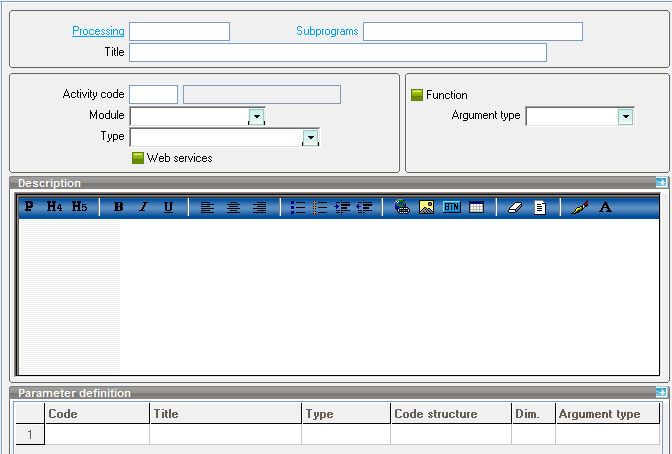3. Load the values as shown below, where the Processing represents the name of the process file created above, and the Subprogram contains the Subprog name in the code.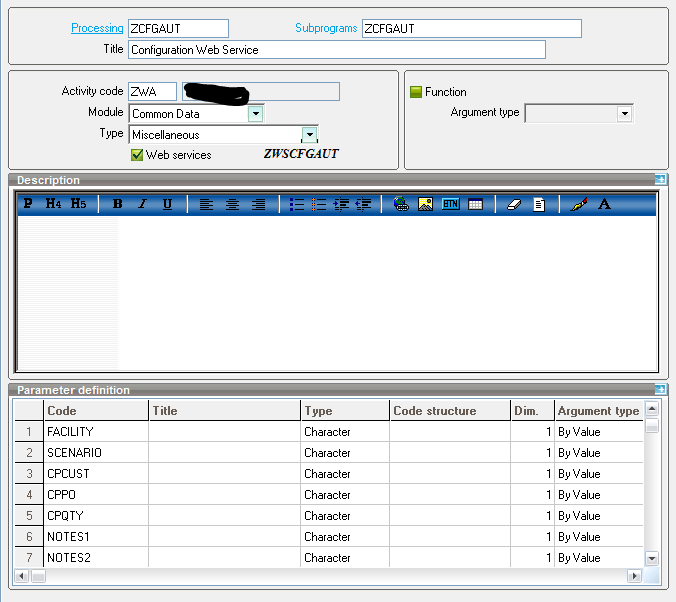4. The parameters will be pulled in automatically based on the parameters in the Subprog definition.
5. Click the "Create" button at the bottom, then click the "Publication" button to generate the Web Service.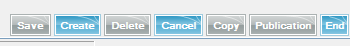6. Enter a name and description for the web service, then click "Create".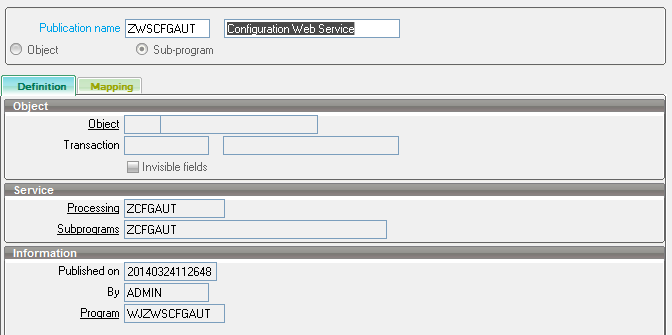## Creating the Web Service

The subprogram created above can be called from other processes within X3, or it can be exposed as a web service. In this particular usage, the subprogram has been exposed as a web service, so it can be called from an external .net application. Once the subprogram has been created, it is fairly straightforward to expose it as a web service.

1. From the Subprograms option above (Development>Processing dictionary>Processings>Subprograms), click the "Publication" button to generate the Web Service.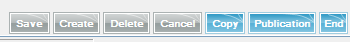2. Enter a name and description for the web service, then click "Create".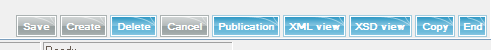The function is now available to be called as a web service. Click the "XML view" to view the parameters for the web service.